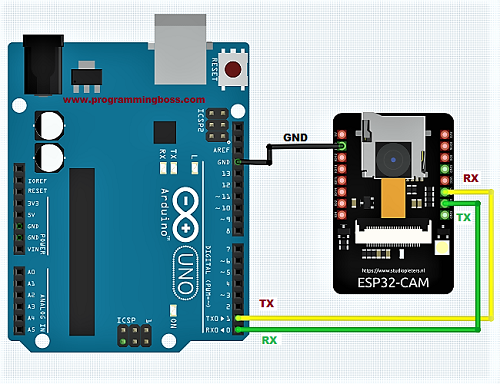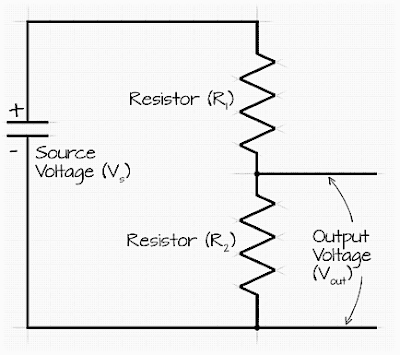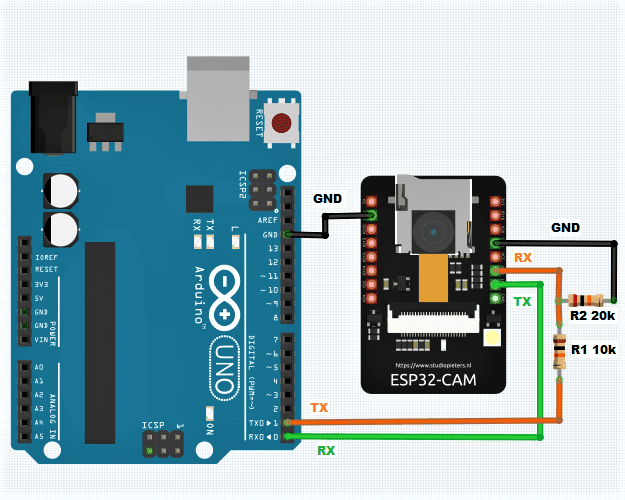# Serial Communication Between Arduino and ESP32 CAM

Serial communication transmits data one bit at a time, sequentially, over a communication channel or computer bus. In the context of Arduino and ESP32, serial communication refers to the transmission of data serially over a single wire or communication line rather than in parallel over multiple wires.Serial communication between Arduino and ESP32 CAM

Two main types of serial communication are commonly used with Arduino and ESP32:

• Asynchronous serial communication: Data is transmitted without a clock signal in asynchronous serial communication. Instead, each data frame's start and stop bits are used to synchronize the communication. Asynchronous serial communication is commonly used for communication over long distances, such as with modems.
• Synchronous serial communication: In synchronous serial communication, a clock signal is used to synchronize data transmission. Synchronous serial communication is typically faster than asynchronous serial communication and is often used for communication between devices on a single circuit board or over short distances.

Both Arduino and ESP32 support both asynchronous and synchronous serial communication. The Arduino Uno has a single hardware serial port (UART) that can be used for serial communication, while the ESP32 has multiple hardware UARTs that can be used for serial communication. Arduino and ESP32 support software-based serial communication using libraries such as SoftwareSerial (for Arduino) and HardwareSerial (for ESP32).

### Circuit diagram of Serial communication between Arduino and ESP32:

The voltage levels of the Arduino Uno and ESP32 are not compatible with direct communication without some form of voltage level shifting. The Arduino Uno operates at 5 volts, while the ESP32 operates at 3.3 volts. If you connect the RX and TX pins of the Arduino Uno and ESP32 directly, you could damage the ESP32 due to the higher voltage.

One way to safely establish serial communication between the Arduino Uno and ESP32 is to use a voltage divider circuit to step down the voltage from the Arduino Uno to a level compatible with the ESP32. A voltage divider is a simple circuit that consists of two resistors connected in series. The voltage at the center point between the two resistors is given by:

Vout = Vin * R2 / (R1 + R2)

To use a voltage divider to step down the voltage from the Arduino Uno to the ESP32, you would connect the Arduino Uno's RX pin to one end of the voltage divider and the ESP32's RX pin to the other end. You would then connect the center point of the voltage divider to the ESP32's RX pin.

Here is an example circuit that demonstrates how you can use a voltage divider to establish serial communication between an Arduino Uno and an ESP32:Voltage divider

In this circuit, R1 is a 10K ohm resistor, and R2 is a 4.7K ohm resistor. This results in a voltage of 3.3 volts at the center point between the two resistors. This voltage is compatible with the ESP32 and can be used to establish serial communication between the Arduino Uno and ESP32.

Keep in mind that you will need to connect the TX pin of the Arduino Uno to the TX pin of the ESP32 similarly, using a voltage divider to step down the voltage from the ESP32 to a level that is compatible with the Arduino Uno.

After implementing voltage divider in between Arduino TX and ESP32 RX, the circuit will look like following:Arduino ESP32 Serial Communication with Voltage Divider

### Code for ESP32 CAM:

Here is an example of how you can establish serial communication between an Arduino Uno and an ESP32-CAM:

#include <SoftwareSerial.h> // include the SoftwareSerial library
const int esp32_rxPin = 2; // RX pin for ESP32-CAM
const int esp32_txPin = 3; // TX pin for ESP32-CAM
SoftwareSerial esp32(esp32_rxPin, esp32_txPin); // create a SoftwareSerial object for the ESP32-CAM
void setup() {
Serial.begin(9600); // start serial communication with the Arduino Uno at 9600 baud
esp32.begin(9600); // start serial communication with the ESP32-CAM at 9600 baud
// configure the ESP32-CAM
esp32.println("AT+CIPMUX=1"); // enable multiple connections
esp32.println("AT+CIPSERVER=1,80"); // start a server on port 80
}
void loop() {
// check if there is data available from the ESP32-CAM
if (esp32.available()) {
// read the data from the ESP32-CAM
Serial.println(data); // print the data to the Arduino Uno's serial monitor
}
// check if there is data available from the Arduino Uno
if (Serial.available()) {
// read the data from the Arduino Uno
esp32.println(data); // send the data to the ESP32-CAM
}
}code-box

This example creates a SoftwareSerial object for the ESP32-CAM and starts a server on port 80. It then continuously checks for data from the ESP32-CAM or the Arduino Uno and sends any data received to the other device.

You will need to include the SoftwareSerial library in your sketch and connect the RX and TX pins of the ESP32-CAM to digital pins 2 and 3 on the Arduino Uno, respectively.

It's important to note that the baud rate, the speed at which the data is transmitted, must be the same on both the Arduino and the ESP32 CAM for the communication to work correctly.

### The video documentation on Arduino and ESP32 Serial Communication

Here is a YouTube video that demonstrates how to establish serial communication between the Arduino and the ESP32 using the Arduino IDE: